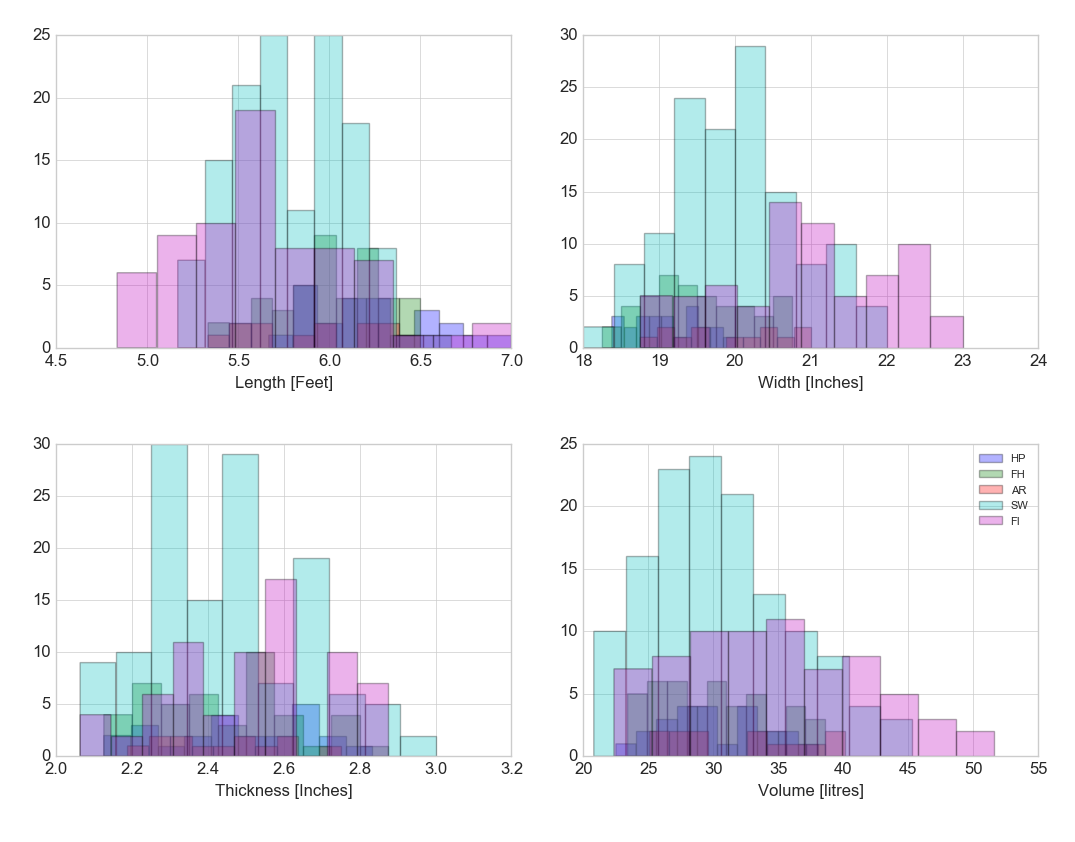## Group of histograms

Date: April 6th 2016
Last updated: February 21st 2017

The data for this example is extracted from a mysql database (not shown here). See analysis/analysis/multiple regression. Then, it is arranged in a pandas dataframe.

Split data

``````# Exploratory plotting
from matplotlib import pyplot as plt
import numpy as np
import math

label_dict = {1: 'HP',
2: 'FH',
3: 'AR',
4: 'SW',
5: 'FI'}

feature_dict = {0: 'Length [Feet]',
1: 'Width [Inches]',
2: 'Thickness [Inches]',
3: 'Volume [litres]'}

with plt.style.context('seaborn-whitegrid'):
plt.figure(figsize=(8, 6))
# loop over the four measurements
for cnt in range(4):
# create a two by two plot frame
# specify each plot with cnt
plt.subplot(2, 2, cnt+1)
for lab in ('HP', 'FH', 'AR', 'SW', 'FI'):
# y==lab returns an array of true or false
# cnt specifies the column to return in x
# returns a vector for each column where
# lab returns true
plt.hist(x[y==lab, cnt],
label=lab,
bins=10,
alpha=0.3,)
plt.xlabel(feature_dict[cnt])
plt.legend(loc='upper right', fancybox=True, fontsize=8)
plt.tight_layout()
plt.show()
``````

Histograms